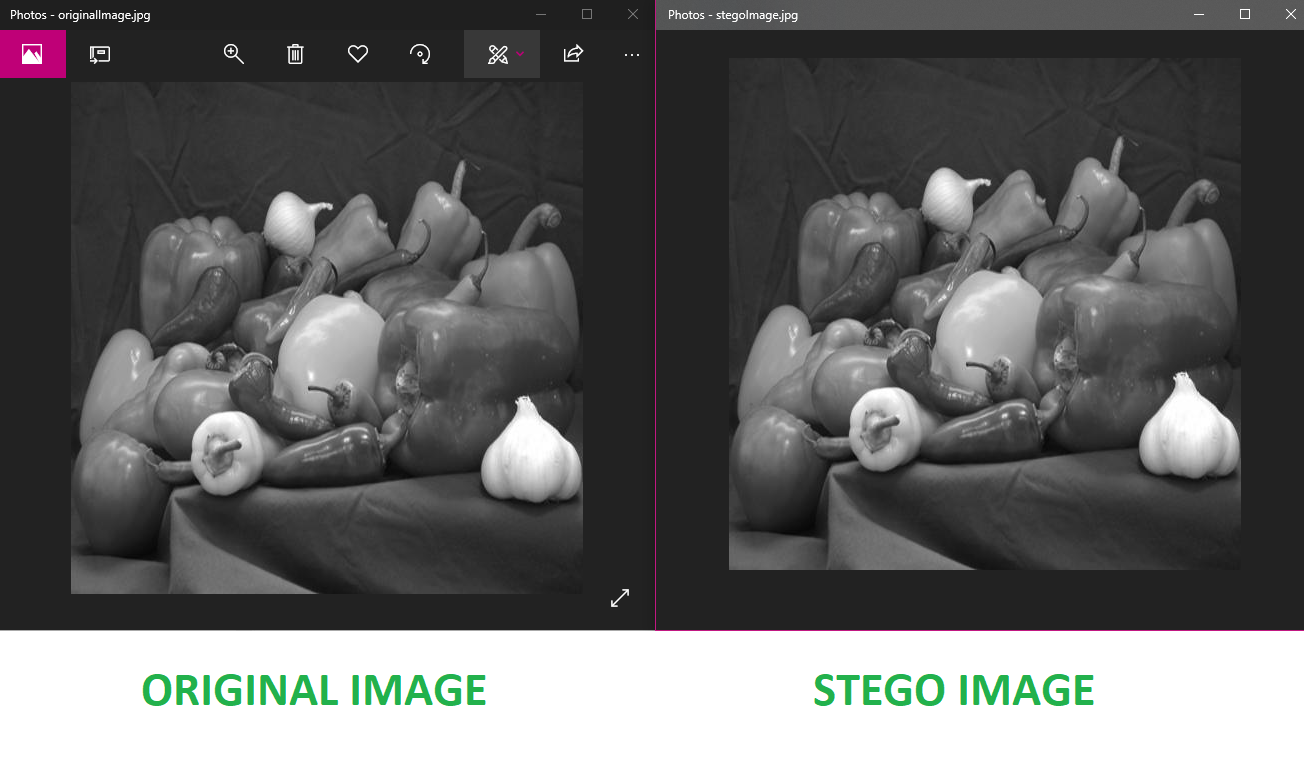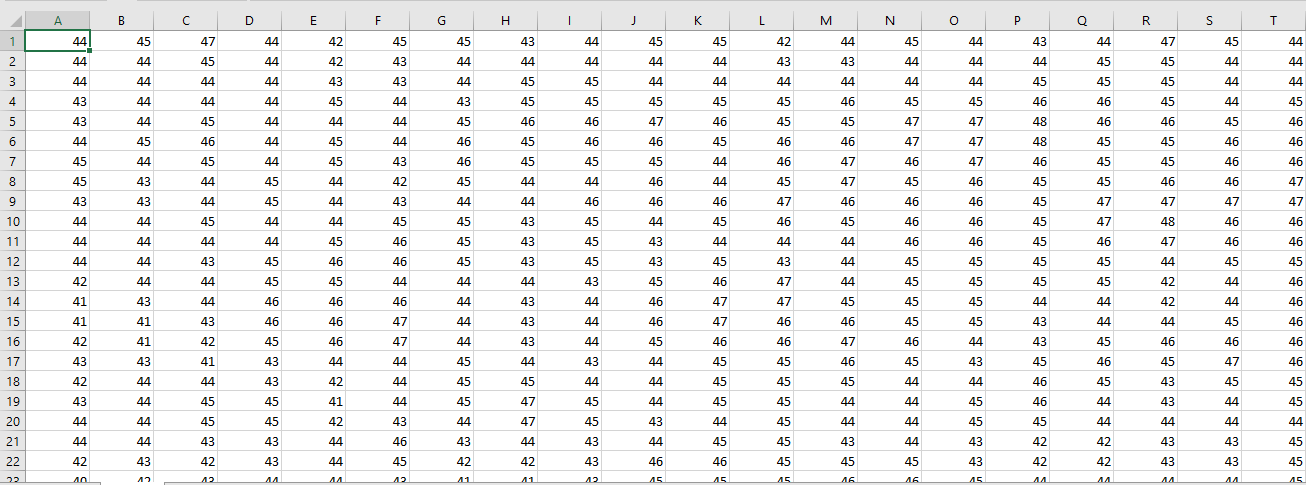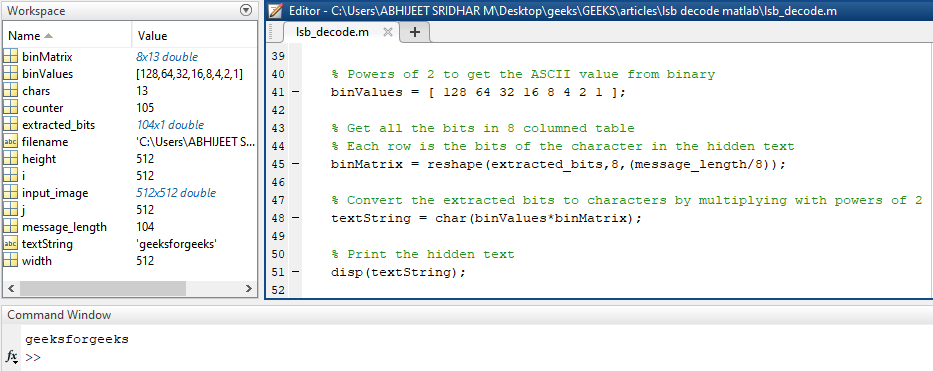# Text extraction from image using LSB based steganography

Prerequisite: LSB based Image steganography using MATLAB

In LSB based Image steganography using MATLAB, we saw how to hide text inside an image. In this article, we are going to see given the stego image or the pixel values and the length of the text embedded as input, how to extract the text from it.

Extraction Process:
The extraction process is simple. We need to first calculate how many pixels is the text stored in. For example, the text “geeksforgeeks” has 13 characters. Each character is represented in 8 bits. So, the number of pixels in which the text is stored will be 13 * 8 = 104. Now after knowing this, we need to traverse through the image, one pixel at a time. We store the Least Significant Bit (LSB) of each pixel in an array extracted_bits. After extracting the LSBs of the required pixels, we need to take every 8 bits from extracted_bits and convert it to the corresponding character. In this way, the text stored in the stego image can be extracted.

In this article, we take the pixel values of the image obtained in the prerequisite article. The values are stored in xlsx format. The message embedded in the image is “geeksforgeeks”.

Note: To store the output image pixels into `.xlsx` format, add the following lines of code to the end of the previous code:

 `filename = ``'path_to_folder\output_img.xlsx'``; ` ` `  `xlswrite(filename, output); `

Here is a screenshot of the input image and the stego image obtained from the prerequisite article:Input : A screenshot of the pixel values of the image:Output : geeksforgeeks

The input file in xlsx format is given here: input_image.xlsx

Below is the implementation in MATLAB:

 `% Clear the existing workspace ` `clear all; ` ` `  `% Clear the command window ` `clc; ` ` `  `% Getting the input image   ` `filename = ``'path_to_folder\output_img.xlsx'``; ` `input_image = xlsread(filename); ` ` `  `% Get height and width for traversing through the image ` `height = size(input_image, 1); ` `width = size(input_image, 2); ` ` `  `% Number of characters of the hidden text ` `chars = 13; ` ` `  `% Number of bits in the message ` `message_length = chars * 8; ` ` `  `% counter to keep track of number of bits extracted ` `counter = 1; ` ` `  `% Traverse through the image ` `for` `i = 1 : height ` `    ``for` `j = 1 : width ` `         `  `        ``% If more bits remain to be extracted ` `        ``if` `(counter <= message_length) ` `             `  `            ``% Store the LSB of the pixel in extracted_bits ` `            ``extracted_bits(counter, 1) = mod(double(input_image(i, j)), 2); ` `             `  `            ``% Increment the counter ` `            ``counter = counter + 1; ` `        ``end` `    ``end` `end` ` `  `% Powers of 2 to get the ASCII value from binary ` `binValues = [ 128 64 32 16 8 4 2 1 ]; ` ` `  `% Get all the bits in 8 columned table ` `% Each row is the bits of the character  ` `% in the hidden text ` `binMatrix = reshape(extracted_bits, 8,  ` `                  ``(message_length/8)); ` ` `  `% Convert the extracted bits to characters ` `% by multiplying with powers of 2 ` `textString = char(binValues*binMatrix);  ` ` `  `% Print the hidden text ` `disp(textString); `

Output:As we can see the output, the text was extracted from the pixel values and result was diplayed to the command window.

Attention reader! Don’t stop learning now. Get hold of all the important CS Theory concepts for SDE interviews with the CS Theory Course at a student-friendly price and become industry ready.

My Personal Notes arrow_drop_upCheck out this Author's contributed articles.

If you like GeeksforGeeks and would like to contribute, you can also write an article using contribute.geeksforgeeks.org or mail your article to contribute@geeksforgeeks.org. See your article appearing on the GeeksforGeeks main page and help other Geeks.

Please Improve this article if you find anything incorrect by clicking on the "Improve Article" button below.

Article Tags :
Practice Tags :

2

Please write to us at contribute@geeksforgeeks.org to report any issue with the above content.